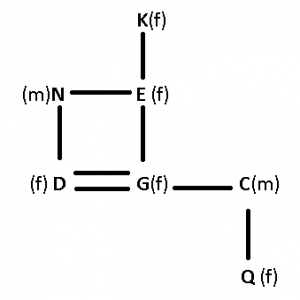# Blood Relation Questions for RBI Grade B

0
2485

Blood Relation Questions for RBI Grade B:

Instructions: Study the information carefully and answer the questions that follow:
Lavkush is the husband of Devi. Lavkush has 3 children. Navin is the brother of Chang and Praneet. Praneet is the daughter of Lavkush.

Question 1: How is Devi related to Chang?
a) Sister
b) Daughter
c) Granddaughter
d) Mother
e) Can’t be determined

Question 2: How is Chang related to Devi?
a) Daughter
b) Son
c) Sister
d) Mother
e) Can’t be determined

Instructions: If A+B means “A is the husband of B”
A-B means “A is the sister of B”
A*B means “A is the brother of B”
A$B means “A is the father of B” Question 3: If A+B-C*D is true, how is A related to D? a) Cousin b) Father c) Brother d) Brother-in-law e) none of these Question 4: If P$Q*R+S is true, how is R related to P?
a) Father-in-law
b) Son
c) Daughter-in-law
d) Son-in-law
e) None of these

Instructions: Study the following information and answer the given questions.
• D is daughter of N. E is wife of N.
• G is sister of D. C is married to G.
• N has no son. K is mother of E.
• Q is only daughter of C.

Question 5: How Q is related to D ?
a) Daughter
b) Cousin
c) Niece
d) Sister-in-law
e) Cannot be determined

Question 6: How N is related to K ?
a) Brother-­in-­law
b) Cousin
c) Son-­in-­law
d) Sister
e) Brother

Question 7: How many daughters N have ?
a) One
b) Three
c) Two
d) Cannot be determined
e)None of these

Question 8: T is the father of M and P. P is the only daughter of V. M is married to N. A and B are children of M. How is V related to B ?
a) Grandmother
b) Uncle
c) Aunt
d) Sister
e) Grandfather

Question 9: Read the following information carefully and answer the question which follows : A is the brother of B. B is the sister of T. T is the mother of P. If it is provided that R is the grandfather of P, how would T be related to R ?
a) Daughter
b) Granddaughter
c) Sister
d) Wife
e) Daughter or Daughter-in-law

Praneet is the daughter of Lavkush. Chang, Praneet and Navin are siblings. So, Chang, Naveen and Praneet are the children of Lavkush and Devi. So, Devi is Sam’s mother.

We can’t identify the genders of the children of Devi by their names. Hence, we don’t know if Chang is Devi’s son or daughter.

A+B-C*D ==> A is the husband of B, B is the sister of C, C is the brother of D.
==>A is the brother-in-law of D.
So correct option to choose is D.

P\$Q*R+S
==>P is the father of Q, Q is a brother of R, R is the husband of S.
==>R is a son of P.
The correct option to choose is B.

D is daughter of N, and sister of G, and E is wife of N, => N is husband of E, and they have two daughters D & G.
C is husband of G having one daughter Q. K is mother of E.
The flow chart will be :
where, (m) represents male
(f) represents female
— represents married couple
= represents siblings
| represents childrenQ is daughter of D’s sister, => Q is the niece of D.
Ans – (C)

D is daughter of N, and sister of G, and E is wife of N, => N is husband of E, and they have two daughters D & G.
C is husband of G having one daughter Q. K is mother of E.
The flow chart will be :
where, (m) represents male
(f) represents female
— represents married couple
= represents siblings
| represents childrenN is the husband of K’s daughter, => N is son-in-law of K.
Ans – (C)

D is daughter of N, and sister of G, and E is wife of N, => N is husband of E, and they have two daughters D & G.
C is husband of G having one daughter Q. K is mother of E.
The flow chart will be :
where, (m) represents male
(f) represents female
— represents married couple
= represents siblings
| represents childrenN has two daughters : D & G
Ans – (C)

T is the father of M and P. P is the only daughter of V.
=> T is the husband of V and P is the sister of M.
M is married to N. A and B are children of M.
Thus, V is grandmother of B.
=> Ans – (A)